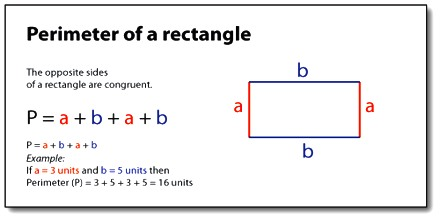Calculating the Perimeter of a Rectangle

Perimeter of a Rectangle is the total distance around the outside of a rectangle. The perimeter of a rectangle is equal to the sum of all the sides. However, since a rectangle’s opposite sides are congruent, we only need to know the length and width.

The perimeter of a rectangle is the distance around the outside of the rectangle. A rectangle has four sides with opposite sides being congruent. The formula for finding the perimeter is Side A + Side B + Side A + Side B. This could also be stated as 2*Side A + 2*Side B or 2*(Side A + Side B)

Or as a formula:

Perimeter = 2 (w + h)

where: w is the width of the rectangle and h is the height of the rectangleExample: Find the perimeter of a rectangular field of length 45 m and width 35 m.

Solution: Perimeter = 2 (w + h)  = 2 (45 + 35)

So, the perimeter is 160 m.